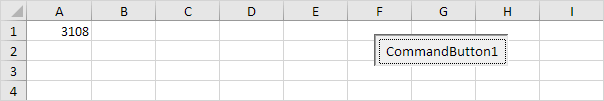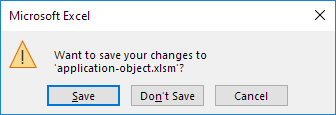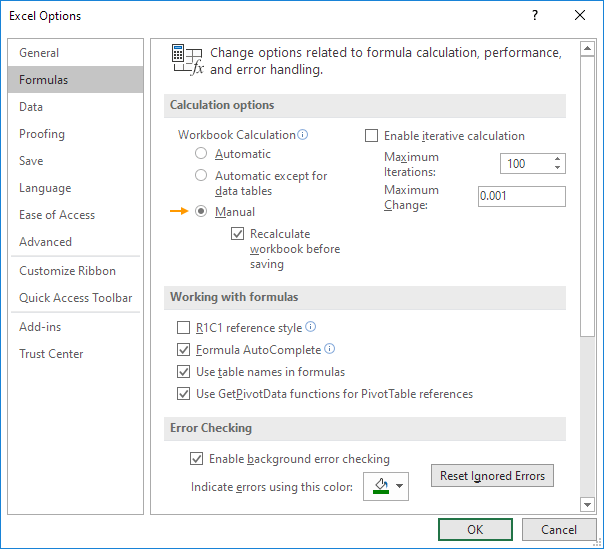# Application Object

The mother of all objects is Excel itself. We call it the Application object. The application object gives access to a lot of Excel related options.

### WorksheetFunction

You can use the WorksheetFunction property in Excel VBA to access Excel functions.

1. For example, place a command button on your worksheet and add the following code line:

Range("A3").Value = Application.WorksheetFunction.Average(Range("A1:A2"))

When you click the command button on the worksheet, Excel VBA calculates the average of the values in cell A1 and cell A2 and places the result into cell A3.Note: instead of Application.WorksheetFunction.Average, simply use WorksheetFunction.Average. If you look at the formula bar, you can see that the formula itself is not inserted into cell A3. To insert the formula itself into cell A3, use the following code line:

Range("A3").Value = "=AVERAGE(A1:A2)"

### ScreenUpdating

Sometimes you may find it useful to disable screen updating (to avoid flickering) while executing code. As a result, your code will run faster.

1. For example, place a command button on your worksheet and add the following code lines:

Dim i As Integer

For i = 1 To 10000
Range("A1").Value = i
Next i

When you click the command button on the worksheet, Excel VBA displays each value a tiny fraction of a second and this can take some time.2. To speed up the process, update the code as follows.

Dim i As Integer

Application.ScreenUpdating = False

For i = 1 To 10000
Range("A1").Value = i
Next i

Application.ScreenUpdating = True

As a result, your code will run much faster and you will only see the end result (10000).

You can instruct Excel VBA not to display alerts while executing code.

1. For example, place a command button on your worksheet and add the following code line:

ActiveWorkbook.Close

When you click the command button on the worksheet, Excel VBA closes your Excel file and asks you to save the changes you made.2. To instruct Excel VBA not to display this alert while executing code, update the code as follows.

ActiveWorkbook.Close

As a result, Excel VBA closes your Excel file, without asking you to save the changes you made. Any changes are lost.

### Calculation

By default,  calculation is set to automatic. As a result, Excel recalculates the workbook automatically each time a value affecting a formula changes. If your workbook contains many complex formulas, you can speed up your macro by setting calculation to manual.

1. For example, place a command button on your worksheet and add the following code line:

Application.Calculation = xlCalculationManual

When you click the command button on the worksheet, Excel VBA sets calculation to manual.

2. You can verify this by clicking on File, Options, Formulas.3. Now when you change the value of cell A1, the value of cell B1 is not recalculated.You can manually recalculate the workbook by pressing F9.

4. In most situations, you will set calculation to automatic again at the end of your code. Simply add the following code line to achieve this.

Application.Calculation = xlCalculationAutomatic

Go to Next Chapter: ActiveX Controls